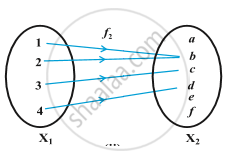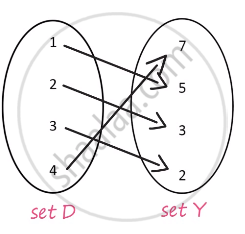# Concept of Functions

• Function, Domain, Co-domain, Range
• Types of function
1. One-one or One to one or Injective function
2. Onto or Surjective function
• Representation of Function
• Graph of a function
• Value of funcation
• Some Basic Functions - Constant Function, Identity function, Power Functions, Polynomial Function, Radical Function, Rational Function, Exponential Function, Logarithmic Function, Trigonometric function

## Definition

A relation f from a set A to a set B is said to be a function if every element of set A has one and only one image in set B.

A function which has either R or one of its subsets as its range is called a real valued function. Further, if its domain is also either R or a subset of R, it is called a real function.

## Notes

In other words, a function f is a relation from a non-empty set A to a non-empty set B such that the domain of f is A and no two distinct ordered pairs in f  have the same first element.
If f is a function from A to B and (a, b) ∈ f, then f (a) = b, where b is called the image of a under f and a is called the preimage of b under f.
The function f from A to B is denoted by f: A → B.
A funcion is a connection between 2 sets A and B f: A→B such that
1) All elements in A are associated to some element in B
2) This association is unique, that means one and only one.
Let's try to understand this with a simple anology,Here, let's say "X"_1 is a set of all children and "X"_2 is a set of all womens. And "X"_1 and "X"_2 have connection as mother and children.
So as per the definition there is a connention between 2 sets "X"_1 and "X"_2 such that all the elements of "X"_1 are associated to some element in set "X"_2 i.e all the childrens are related to a particualr mother, and this association is unique because no one child can have two or more mothers, but a mother can have more than one child.Consider the sets D and Y related to each ther as shown, clearly every element in the set D is related to exactly one element in the set Y. So the given relation is a function. f: D → Y.
Here, D is the domain of the function and Y is the co domain of the function.
f(1)= 5
Here, 5 is called the image of 1 under f and 1 is called preimage of 5 under f.
The range of this function is, Range= {2,3,5,7}
The range is a set of real numbers so the function is Real valued function.

If you would like to contribute notes or other learning material, please submit them using the button below.

### Shaalaa.com

function [00:07:57]
S
0%